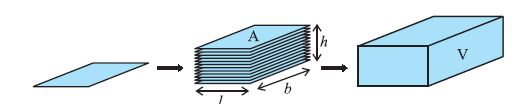Email us to get an instant 20% discount on highly effective K-12 Math & English kwizNET Programs!

#### Online Quiz (WorksheetABCD)

Questions Per Quiz = 2 4 6 8 10

### Geometry6.8 Volume of Cuboid

 The measure of the occupied space is called the Volume of the object. If an object is solid, then the space occupied by such an object is measured and is called the Volume of the object. On the other hand if the object is hollow then the interior is empty and can be filled with air or some liquid then will take the shape of its container. In this case, the volume of the substance that can fill the interior is called the Capacity of the container. In short the volume of the object is the measure of the space it occupies and the capacity of an object is the volume of the substance its interior can accommodate. Hence the unit of measurement of either of the two is cubic unit.In the above figure the area of each rectangle is A and height up to which the rectangles are stacked is h and the volume of the cuboid is V. V = A x h Volume of a Cuboid = length x width x height = base area x height Volume of Cuboid = l x w x h = A x h
 Q 1: A cuboid has volume is 480 cm3 and the width of 8 cm and height of 5cm. What is its length.15 cm25 cm12 cm Q 2: A large box has dimensions 20, 25 and 60cm. Small box has dimensions of 5, 5 and 10 cm. How many of small boxes would fit in the large box.120250200 Q 3: A rectangular tank has dimensions of 35 cm x 30 cm x 20 cm contains water to a height of 15 cm. Find the volume of water in the tank. 15750 cm315500 cm312000 cm3 Q 4: A cuboid has sides of length 5 m, 3 m and 1 m. What is the volume of the cuboid in cm3? 150015000000150000 Q 5: Calculate the volume of a cuboid with length 0.5m, width of 0.1m and height of 0.5m.0.25 m30.025 m32.5 m3 Q 6: Find the volume of the cuboid whose length 4cm, width 2cm and height 7cm.56 cm352 cm345 cm3 Question 7: This question is available to subscribers only! Question 8: This question is available to subscribers only!